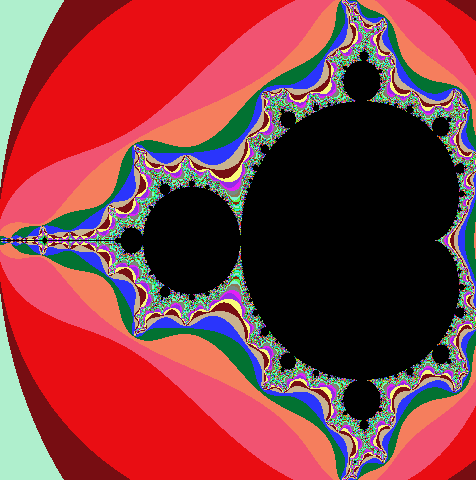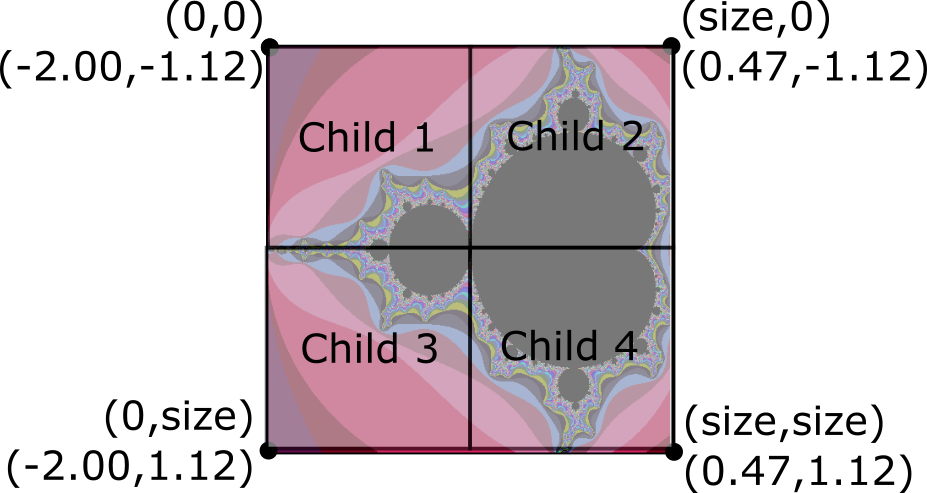## Assignment 9: Many processes make light work

Due in two parts — Friday, April 8 and Friday April 15 — before midnight

The goals for this assignment are:

• Implement an algorithm using multiple processes with fork()

• Compare single- and multi-process implementation performance

• Work with fork(), wait(), process ids, and shared memory

## Mandelbrot Set

The mandelbrot set is fractal popularized by Benoit Mandelbrot in 1980.

The mandelbrot set consists of the set of complex numbers z for which $z^2 + c$ does not diverge to infinity when z starts at 0. To visualize this set, recall that a complex number, $z = x + yi$, can be visualized as a 2D point $(x,y)$. Although the set derives from complex numbers, we can compute the set by thinking about 2D coordinates, $(x,y)$.

To see whether the complex number $(x,y)$ diverges, we simply need a loop that repeatedly computes $z^2 + c$. If we expand the complex number multiplication of $z = x + y*i$, z will change each iteration based on the following algorith. To test for divergence, we check whether z goes out of the bounds of 4*4. If z does go out of bounds, we assign it a color based on how quickly it "escaped" the distance 4*4. If after MAX iterations, z is still smaller than 4*4, it belongs to the set and we color it black.

The last thing we need to draw the set is the region of values for x and y that bound the set. X should vary from -2.0 to 0.47. Y should vary from -1.12 to 1.12. Here is the full algorithm. Assume that the image width and height are the same, e.g. square images.

``````for each row in the image
for each col in the image
xfrac = row / image_size
yfrac = col / image_size
x0 = xmin + xfrac * (xmax - xmin)
y0 = ymin + yfrac * (ymax - ymin)

x = 0
y = 0
iter = 0
while (iter < MAX && x*x + y*y < 2*2)
xtmp = x*x - y*y + x0
y = 2*x*y + y0
x = xtmp
iter++

if (iter < MAX) // escaped
color = palette[iter]
else
color = black

write color to image at location (row,col)``````

### Palette

The palette should contain the same number of colors as MAX iterations. Use `ppm_pixel` to represent colors in the pallet. You can either generate random colors or compute a base color and jitter it e.g.``````for each palette color red = rand() % 255 green = rand() % 255 blue = rand() % 255````````````basered = rand() % 255 basegreen = rand() % 255 baseblue = rand() % 255 for each palette color red = basered + rand() % 100 - 50 green = basegreen + rand() % 100 - 50 blue = baseblue + rand() % 100 - 50``````

## 1. Single

This is due Friday April 8, before midnight

In the file, `single_mandelbrot`, compute a program that outputs a PPM image of the mandelbrot set. You have been given basecode that initializes the following values from command line arguments. This code uses the getopt function.

Arguments:

• `-s <size>` the image width and height

• `-l <xmin>` the leftmost coordinate, e.g. minimum x value

• `-r <xmax>` the rightmost coordinate, e.g. maximum x value

• `-t <ymin>` the topmost coordinate, e.g. minimum y value

• `-b <ymax>` the bottommost coordinate, e.g. maximum y valueWhen you run your program, you should get the output such as the following

``````$make single_mandelbrot$ ./single_mandelbrot
Generating mandelbrot with size 480x480
X range = [-2.0000,0.4700]
Y range = [-1.1200,1.1200]
Computed mandelbrot set (480x480) in 0.323261 seconds
Writing file: mandelbrot-480-1649001071.ppm``````

Requirements and hints:

• You should re-use your PPM functions from A06. If you use a 2D array of arrays, be sure to modify and commit `read_ppm.h`!

• Allocate an array of pixels using malloc and then save the final image using `write_ppm`.

• You should output the number of seconds needed to compute the image. Use this class example, matrix.c for an example.

• Your output filename should have the format `mandelbrot-<size>-<timestamp>.ppm`. The timestamp can be obtained by calling `time(0)`.

• Set a random seed to ensure that the color palette is different each time, e.g. `srand(time(0))`

• In your `README.adoc`, call `single_mandelbrot` with sizes of 100, 400, 800, 1000, and 2000. Record the number of seconds it takes to compute each image.

• (Optional) Experiment with visualizing other regions of the mandelbrot set. For example, if you run with `./single_mandelbrot -s 480 -l -0.02524993 -r 0.00975 -b -0.8172 -t -0.79725`, you get the following image## 2. Multiple

This is due Friday April 15, before midnight

In the file, `multi_mandelbrot`, compute a program that outputs a PPM image of the mandelbrot set using multiple processes spawned with `fork()`. Your basecode implements the same command line arguments in with `single_mandelbrot`.

Your program should use `shared memory` to split the computation of the image across 4 child processes. After each child completes, the parent process should save the result to a file using `write_ppm`.

``````$./multi_mandelbrot Generating mandelbrot with size 480x480 Num processes = 4 X range = [-2.0000,0.4700] Y range = [-1.1200,1.1200] Launched child process: 9150 Launched child process: 9151 9150) Sub-image block: cols (0, 240) to rows (0,240) 9151) Sub-image block: cols (240, 480) to rows (0,240) Launched child process: 9152 9152) Sub-image block: cols (0, 240) to rows (240,480) Launched child process: 9153 9153) Sub-image block: cols (240, 480) to rows (240,480) Child process complete: 9150 Child process complete: 9152 Child process complete: 9151 Child process complete: 9153 Computed mandelbrot set (480x480) in 0.152178 seconds Writing file: multi-mandelbrot-480-1649001405.ppm``````Requirements and hints: • You should re-use your PPM functions from A06. If you use a 2D array of arrays, be sure to modify and commit `read_ppm.h`! • Allocate an array of pixels using `shared memory` and then save the final image using `write_ppm`. Use the example from class as a reference. • Print the pids and work tasks for each child. • Refactor the code from `single_mandelbrot` to compute the mandelbrot set using a function. Then re-use the same function in both `single_mandelbrot.c` and `multi_mandelbrot.c` • Use `fork()` to create 4 child processes and `wait()` to wait for each to complete. • You should output the number of seconds needed to compute the image. Use this class example, matrix.c for an example. • Your output filename should have the format `multi-mandelbrot-<size>-<timestamp>.ppm`. The timestamp can be obtained by calling `time(0)`. • Set a random seed to ensure that the color palette is different each time, e.g. `srand(time(0))` • In your `README.adoc`, call `single_mandelbrot` with sizes of 100, 400, 800, 1000, and 2000. Record the number of seconds it takes to compute each image. ## 3. Submit your work Submit both your code, images, and a brief report containing your results in your README. 1) Push your code work to github ``````$ git status
$git add .$ git status
$git commit -m "assignment complete"$ git status
$git push$ git status``````